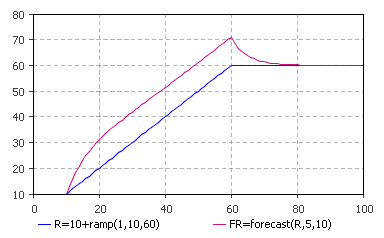AnyLogic

# forecast

forecast(input, averageTime, horizon) is the system dynamics function that returns a forecast of the value the input will take on at averageTime horizon.

The plot below illustrates how the function works:This function can be called in formulas of system dynamics variables and its input units match the output units.

#### Units

input — unit

averageTime — time

horizon — time

forecast() — unit

The output units are the same as the input ones.